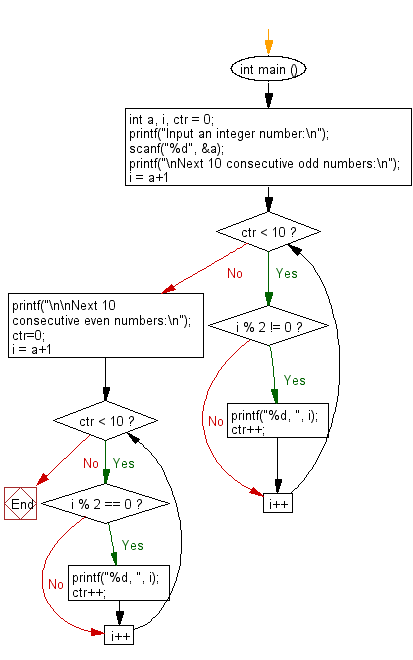﻿ C : Print ten consecutive odd and even numbers

# C Exercises: Accept an integer and print next ten consecutive odd and even numbers

## C Basic Declarations and Expressions: Exercise-107 with Solution

Write a C program that prints ten consecutive odd and even numbers after accepting an integer.

Sample Solution:

C Code:

``````#include <stdio.h>

int main () {
int a, i, ctr = 0;

// Prompt the user to input an integer number
printf("Input an integer number:\n");
scanf("%d", &a);

// Print the next 10 consecutive odd numbers
printf("\nNext 10 consecutive odd numbers:\n");

for (i = a + 1; ctr < 10; i++){

if (i % 2 != 0){

printf("%d, ", i);
ctr++;

}
}

// Reset counter for even numbers
ctr = 0;

// Print the next 10 consecutive even numbers
printf("\n\nNext 10 consecutive even numbers:\n");

for (i = a + 1; ctr < 10; i++){

if (i % 2 == 0){

printf("%d, ", i);
ctr++;

}
}
}
``````

Sample Output:

```Input an integer number:
15

Next 10 consecutive odd numbers:
17, 19, 21, 23, 25, 27, 29, 31, 33, 35,

Next 10 consecutive even numbers:
26, 28, 30, 32, 34, 36, 38, 40, 42, 44,
```

Flowchart:C programming Code Editor:

What is the difficulty level of this exercise?

Test your Programming skills with w3resource's quiz.

﻿